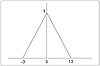# 130.C++ Neural Networks and Fuzzy Logic by Valluru B. Rao M&T Books, IDG Books Worldwide, Inc. ISBN: 1558515526   Pub Date: 06/01/95

#### Fuzzy Numbers

A fuzzy number is an ordered pair of numbers (a, b) with respect to a reference function L, which gives you the membership function. Here b has to be a positive number.

Here are the properties of L, the reference function:

1.  It is a function of one variable and is symmetric about 0. That is, L(x) = L(-x).
2.  It has a value of 1 at x = 0. In other words, L(0) = 1.
3.  It is generally decreasing, when x is 0 or a positive number, meaning that its value drops as the value of its argument is increased. For example, L(2) < L(1). Thus L(x) has its values less than 1 for positive values of x. It does not make sense to have negative numbers as values of L, and so you ignore the values of x that cause such values for L.
4.  The maximum value for the function is 1, at x = 0. It has a sort of a bell-shaped curve.

If A is a fuzzy number with the ordered pair (a, b) with b > 0, and if the reference function is L, you do the following:

You write the fuzzy number as A = (a, b)L. You get the membership of any element x, by taking the quantity (x - a) / b (which reminds you of how you get a z-score), and evaluate the reference function L at this argument. That is:

`      mA(x) = L( (x-a) / b) `

Examples of a reference function L are:

Example 1: L(x) = max ( 0, 1- x2)

You obtain the following shown in Table 16.8.

Table 16.8 Reference Function L

x L(x)
-2 0
-1 0
-0.5 0.75
0 1
0.5 0.75
1 0
2 0

This function is not strictly decreasing though. It remains a constant at 0 for x > 1.

Example 2: L(x) = 1/ (1 + x2 )

You get the values shown in Table 16.9.

Table 16.9 Reference Function L

x L(x)
-7 0.02
-2 0.2
-1 0.5
-0.5 0.8
0 1
0.5 0.8
1 0.5
2 0.2
7 0.02

Let us now determine the membership of 3 in the fuzzy number A = (4, 10)L, where L is the function in the second example above, viz., 1/ (1 + x2).

First, you get (x- 4) / 10 = (3 - 4) / 10 = - 0.1. Use this as the argument of the reference function L. 1/ (1 + (- 0.1)2 ) gives 0.99. This is expressed as follows:

`      mA(3) = L( (3-4) / 10) = 1/ (1 + (- 0.1)2 ) = 0.99 `

You can verify the values, mA(0) = 0.862, and mA(10) = 0.735.

#### Triangular Fuzzy Number

With the right choice of a reference function, you can get a symmetrical fuzzy number A, such that when you plot the membership function in A, you get a triangle containing the pairs (x, mA(x)), with mA(x) > 0. An example is A = (5, 8)L, where L = max(1 - |x|, 0).

The numbers x that have positive values for mA(x) are in the interval ( -3, 13 ). Also, mA( -3 ) = 0, and mA(13) = 0. However, mA(x) has its maximum value at x = 5. Now, if x is less than -3 or greater than 13, the value of L is zero, and you do not consider such a number for membership in A. So all the elements for which membership in A is nonzero are in the triangle.

This triangular fuzzy number is shown in Figure 16.1. The height of the triangle is 1, and the width is 16, twice the number 8, midpoint of the base is at 5. The pair of numbers 5 and 8 are the ones defining the symmetrical fuzzy number A. The vertical axis gives the membership, so the range for this is from 0 to 1.Figure 16.1  Triangular membership function.

#### Linear Possibility Regression Model

Assume that you have (n + 1)-tuples of values of x1, ... xn, and y. That is, for each i, i ranging from 1 to k, you have (x1, ... , xn, y), which are k sample observations on X1, ... , Xn, and Y. The linear possibility regression model is formulated differently depending upon whether the data collected is crisp or fuzzy.

Let us give such a model below, for the case with crisp data. Then the fuzziness lies in the coefficients in the model. You use symmetrical fuzzy numbers, Aj = (aj, bj)L. The linear possibility regression model is formulated as:

`      Yj = A0 + A1Xj1 + ... + AnXjn `

The value of Y from the model is a fuzzy number, since it is a function of the fuzzy coefficients. The fuzzy coefficients Ajare chosen as those that minimize the width (the base of the triangle) of the fuzzy number Yj. But Aj is also determined by how big the membership of observed yj is to be, in the fuzzy number Yj . This last observation provides a constraint for the linear programming problem which needs to be solved to find the linear possibility regression. You select a value d, and ask that mY(y) [ge] d.

We close this section by observing that linear possibility regression gives triangular fuzzy numbers for Y, the dependent variable. It is like doing interval estimation, or getting a regression band. Readers who are seriously interested in this topic should refer to Terano, et al. (see references).C++ Neural Networks and Fuzzy Logic
ISBN: 1558515526
EAN: 2147483647
Year: 1995
Pages: 139

Similar book on Amazon